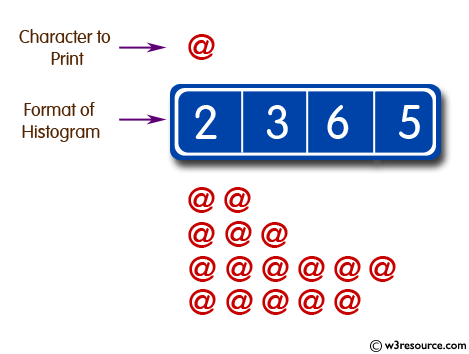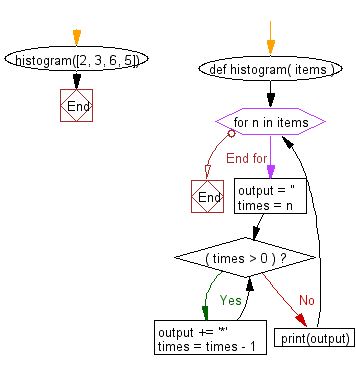﻿ Python: Create a histogram from a given list of integers - w3resource# Python: Create a histogram from a given list of integers

## Python Basic: Exercise-26 with Solution

Write a Python program to create a histogram from a given list of integers.

Pictorial Presentation:Sample Solution:-

Python Code:

``````def histogram( items ):
for n in items:
output = ''
times = n
while( times > 0 ):
output += '*'
times = times - 1
print(output)

histogram([2, 3, 6, 5])
```
```

Sample Output:

```**
***
******
*****
```

Flowchart:## Visualize Python code execution:

The following tool visualize what the computer is doing step-by-step as it executes the said program:

Python Code Editor:

Have another way to solve this solution? Contribute your code (and comments) through Disqus.

What is the difficulty level of this exercise?

Test your Python skills with w3resource's quiz

﻿

## Python: Tips of the Day

Try-catch-else construct:

```try:
foo()
except Exception:
print("Exception occured")
else:
print("Exception didnt occur")
finally:
print("Always gets here")
```